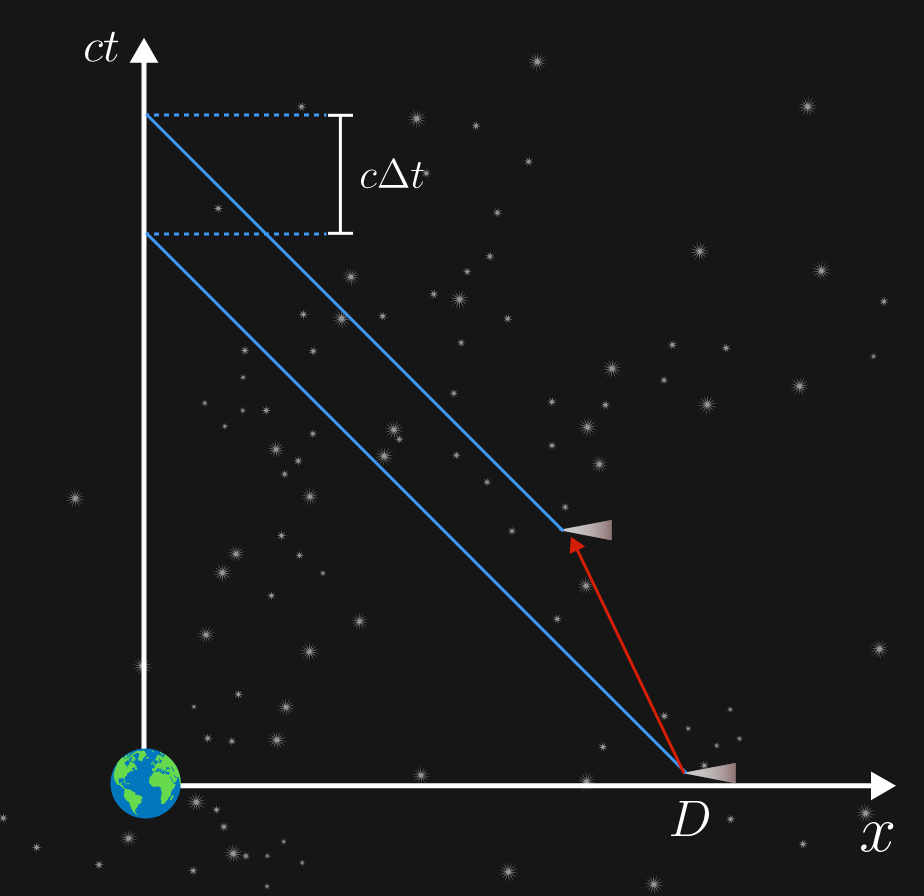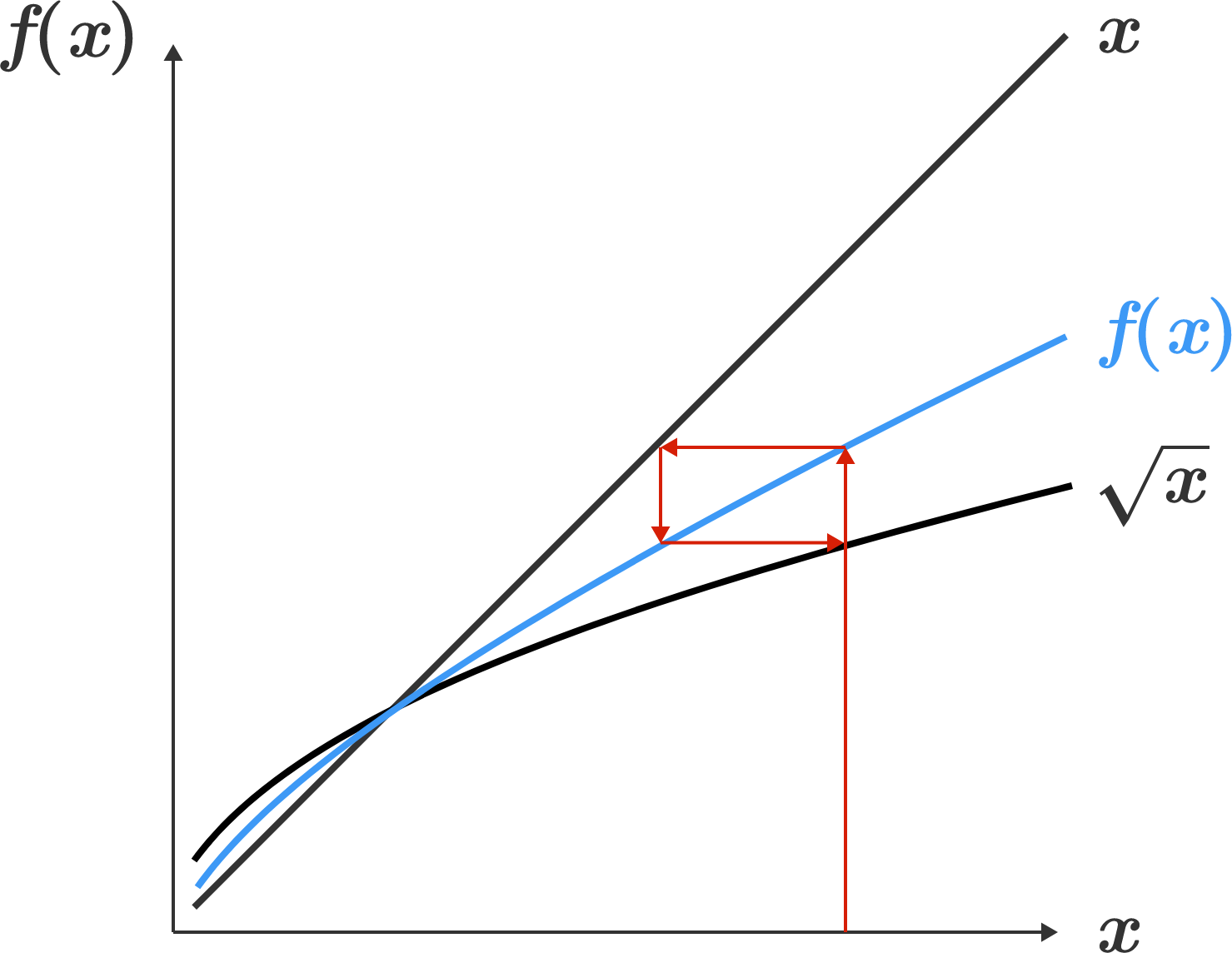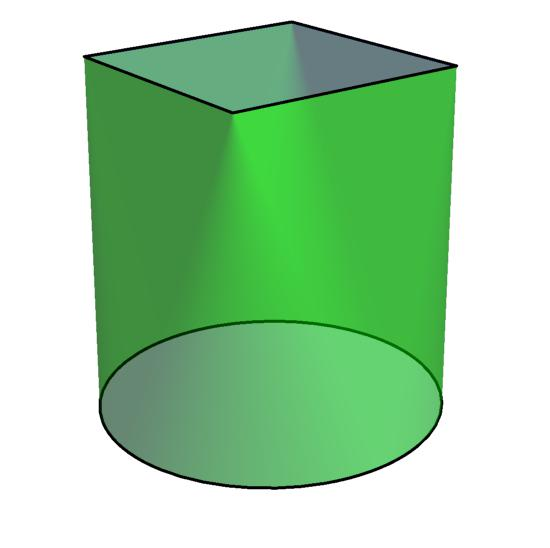# Problems of the Week

Contribute a problemA spacecraft approaching Earth very quickly is observed at a distance of $D.$ This has never happened before, so you check your instruments for time $\Delta t,$ and then you check the position of the craft again.

Now you can see it at a distance of $D- 3 c \Delta t,$ where $c$ is the speed of light.

The craft is traveling at a constant speed of $v$ directly toward Earth. What is $v?$

Assume you have made your measurements from an inertial frame very close to Earth.


Hint: The correct solution requires no transformation.

Let $f: \mathbb{R}^+ \to \mathbb{R}^+$ be a positive, monotonically increasing function with the property $f\big(f(x)\big) = \sqrt{x}.$ What is the value $f (2)$?Eight people are sitting around a circular table. In how many ways can they reseat themselves so that nobody is seated to the immediate right of the same person as before?

As an explicit example, if they were originally seated as follows: $\quad \, \, \ \text{A} \, \, \quad \\ \quad \text{H} \quad \, \, \ \ \ \ \text{B} \quad \\ \text{G} \quad \quad \quad \, \, \ \text{C} \\ \quad \text{F} \quad \, \, \ \ \ \ \text{D} \quad \\ \quad \, \, \ \text{E} \, \, \quad \\$ then they can reseat themselves as follows, and no one would be sitting to the right of the same person as before: $\quad \, \, \ \text{A} \, \, \quad \\ \quad \text{B} \quad \, \, \ \ \ \ \text{H} \quad \\ \text{C} \quad \quad \quad \, \, \ \text{G} \\ \quad \text{D} \quad \, \, \ \ \ \ \text{F} \quad \\ \quad \, \, \ \text{E} \, \, \quad \\$ Details and Assumptions:

• Arrangements that are rotations of each other are considered the same.
• Arrangements that are reflections of each other are considered distinct.

A computable number is one which, given enough time, can be calculated to whatever degree of precision is desired. For example, the irrational number which has a 1 in every prime slot $0.01101010001010001 \ldots$ is computable. To calculate it to an accuracy of $10^{-n}$, we just need to determine which of the first $n$ positive integers are prime.

Are there countably many or uncountably many computable numbers?Define a ruled prism to be a solid with 2 parallel faces and a ruled surface joining the 2 parallel faces continuously and without overlap.

Given that you know the perpendicular distance between the 2 parallel faces and the areas of $n$ cross-sections of the ruled prism, what is the minimum $n$ such that you can know the exact volume of the ruled prism?

(You can choose which cross-sections to find the area of.)

The diagram above is an example of a ruled prism with a square and a circle as the parallel faces and a ruled surface connecting them.

×